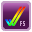# Option: uae_chipset_compatible

```Description: Enable default chipset features for a specific model
Default:
Example: -
Type: choice

Allows you to specify what Amiga model you want to emulate with regards to
chipset features. Normally, you do not have to use this option, as it is
automatically set (corresponding to the chosen Amiga model).

Value: -
Value: Generic
Value: CDTV
Value: CD32
Value: A500
Value: A500+
Value: A600
Value: A1000
Value: A1200
Value: A2000
Value: A3000
Value: A3000T
Value: A4000
Value: A4000T

In some cases, you may have to set it to - in order to allow modifying
chipset features via other uae_* options.

FS-UAE: Setting it to - will still cause the chipset to be configured
correctly initially, it just prevents FS-UAE from resetting the chipset
options after they have been modified with other uae_* options.

Code:

if c.uae_chipset_compatible.explicit:
value = c.uae_chipset_compatible.explicit
elif c.amiga_model == "A500":
value = "A500"
elif c.amiga_model == "A500/512K":
value = "A500"
elif c.amiga_model == "A500+":
value = "A500+"
elif c.amiga_model == "A600":
value = "A600"
elif c.amiga_model == "A1000":
value = "A1000"
elif c.amiga_model == "A1200":
value = "A1200"
elif c.amiga_model == "A1200/3.0":
value = "A1200"
elif c.amiga_model == "A1200/020":
value = "A1200"
elif c.amiga_model == "A1200/1230":
value = "A1200"
elif c.amiga_model == "A1200/1240":
value = "A1200"
elif c.amiga_model == "A1200/1260":
value = "A1200"
elif c.amiga_model == "A1200/PPC":
value = "A1200"
elif c.amiga_model == "A3000":
value = "A3000"
elif c.amiga_model == "A4000":
value = "A4000"
elif c.amiga_model == "A4000/040":
value = "A4000"
elif c.amiga_model == "A4000/OS4":
value = "A4000"
elif c.amiga_model == "A4000/PPC":
value = "A4000"
elif c.amiga_model == "CD32":
value = "CD32"
elif c.amiga_model == "CD32/FMV":
value = "CD32"
elif c.amiga_model == "CDTV":
value = "CDTV"
else:
f.fail("Unknown amiga_model")
```